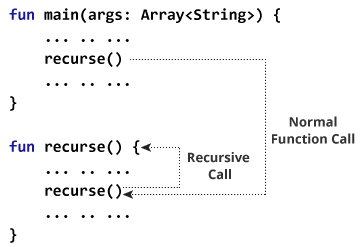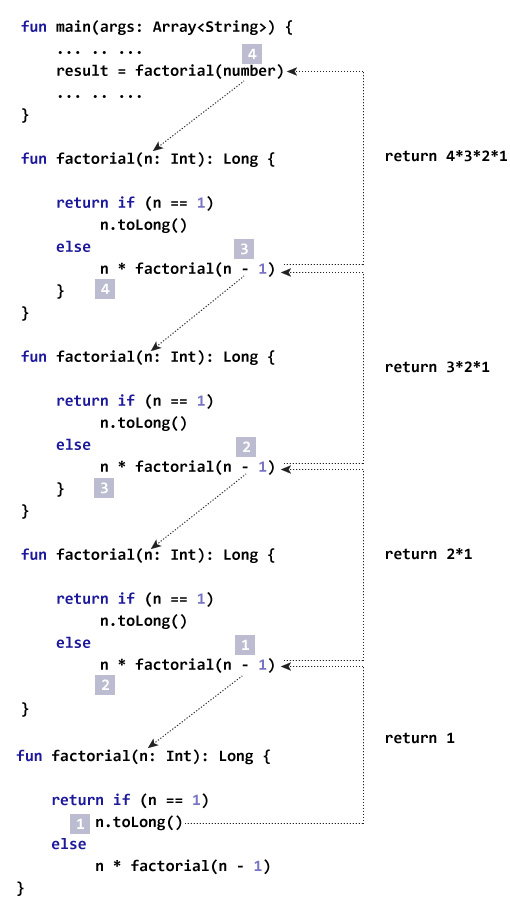Kotlin Recursion (Recursive Function) and Tail Recursion

# Kotlin Recursion (Recursive Function) and Tail Recursion

#### In this article, you will learn to create recursive functions; a function that calls itself. Also, you will learn about tail recursive function.

A function that calls itself is known as recursive function. And, this technique is known as recursion.

A physical world example would be to place two parallel mirrors facing each other. Any object in between them would be reflected recursively.

### How does recursion work in programming?

```fun main(args: Array<String>) {
... .. ...
recurse()
... .. ...
}

fun recurse() {
... .. ...
recurse()
... .. ...
}
```

Here, the `recurse()` function is called from the body of `recurse()` function itself. Here's how this program works:Here, the recursive call continues forever causing infinite recursion.

To avoid infinite recursion, if...else (or similar approach) can be used where one branch makes the recursive call and other doesn't.

### Example: Find factorial of a Number using Recursion

``````fun main(args: Array<String>) {
val number = 4
val result: Long

result = factorial(number)
println("Factorial of \$number = \$result")
}

fun factorial(n: Int): Long {
return if (n == 1) n.toLong() else n*factorial(n-1)
}``````

When you run the program, the output will be:

`Factorial of 4 = 24`

### How this program works?

The recursive call of the `factorial()` function can be explained in the following figure:Here are the steps involved:

```factorial(4)              // 1st function call. Argument: 4
4*factorial(3)            // 2nd function call. Argument: 3
4*(3*factorial(2))        // 3rd function call. Argument: 2
4*(3*(2*factorial(1)))    // 4th function call. Argument: 1
4*(3*(2*1))
24
```

### Kotlin Tail Recursion

Tail recursion is a generic concept rather than the feature of Kotlin language. Some programming languages including Kotlin use it to optimize recursive calls, whereas other languages (eg. Python) do not support them.

### What is tail recursion?

In normal recursion, you perform all recursive calls first, and calculate the result from return values at last (as show in the above example). Hence, you don't get result until all recursive calls are made.

In tail recursion, calculations are performed first, then recursive calls are executed (the recursive call passes the result of your current step to the next recursive call). This makes the recursive call equivalent to looping, and avoids the risk of stack overflow.

### Condition for tail recursion

A recursive function is eligible for tail recursion if the function call to itself is the last operation it performs. For example,

Example 1: Not eligible for tail recursion because the function call to itself `n*factorial(n-1)` is not the last operation.

```fun factorial(n: Int): Long {

if (n == 1) {
return n.toLong()
} else {
return n*factorial(n - 1)
}
}```

Example 2: Eligible for tail recursion because function call to itself `fibonacci(n-1, a+b, a)` is the last operation.

```fun fibonacci(n: Int, a: Long, b: Long): Long {
return if (n == 0) b else fibonacci(n-1, a+b, a)
}
```

To tell compiler to perform tail recursion in Kotlin, you need to mark the function with `tailrec` modifier.

### Example: Tail Recursion

``````import java.math.BigInteger

fun main(args: Array<String>) {
val n = 100
val first = BigInteger("0")
val second = BigInteger("1")

println(fibonacci(n, first, second))
}

tailrec fun fibonacci(n: Int, a: BigInteger, b: BigInteger): BigInteger {
return if (n == 0) a else fibonacci(n-1, b, a+b)
}``````

When you run the program, the output will be:

`354224848179261915075`

This program computes the 100th term of the Fibonacci series. Since, the output can be a very large integer, we have imported BigInteger class from Java standard library.

Here, the function `fibonacci()` is marked with `tailrec` modifier and the function is eligible for tail recursive call. Hence, the compiler optimizes the recursion in this case.

If you try to find the 20000th term (or any other big integer) of the Fibonacci series without using tail recursion, the compiler will throw `java.lang.StackOverflowError` exception. However, our program above works just fine. It's because we have used tail recursion which uses efficient loop based version instead of traditional recursion.

### Example: Factorial Using Tail Recursion

The example to compute factorial of a number in the above example (first example) cannot be optimized for tail recursion. Here's a different program to perform the same task.

``````fun main(args: Array<String>) {
val number = 5
println("Factorial of \$number = \${factorial(number)}")
}

tailrec fun factorial(n: Int, run: Int = 1): Long {
return if (n == 1) run.toLong() else factorial(n-1, run*n)
}```
```

When you run the program, the output will be:

`Factorial of 5 = 120`

The compiler can optimize the recursion in this program as the recursive function is eligible for tail recursion, and we have used `tailrec` modifier that tells compiler to optimize the recursion.